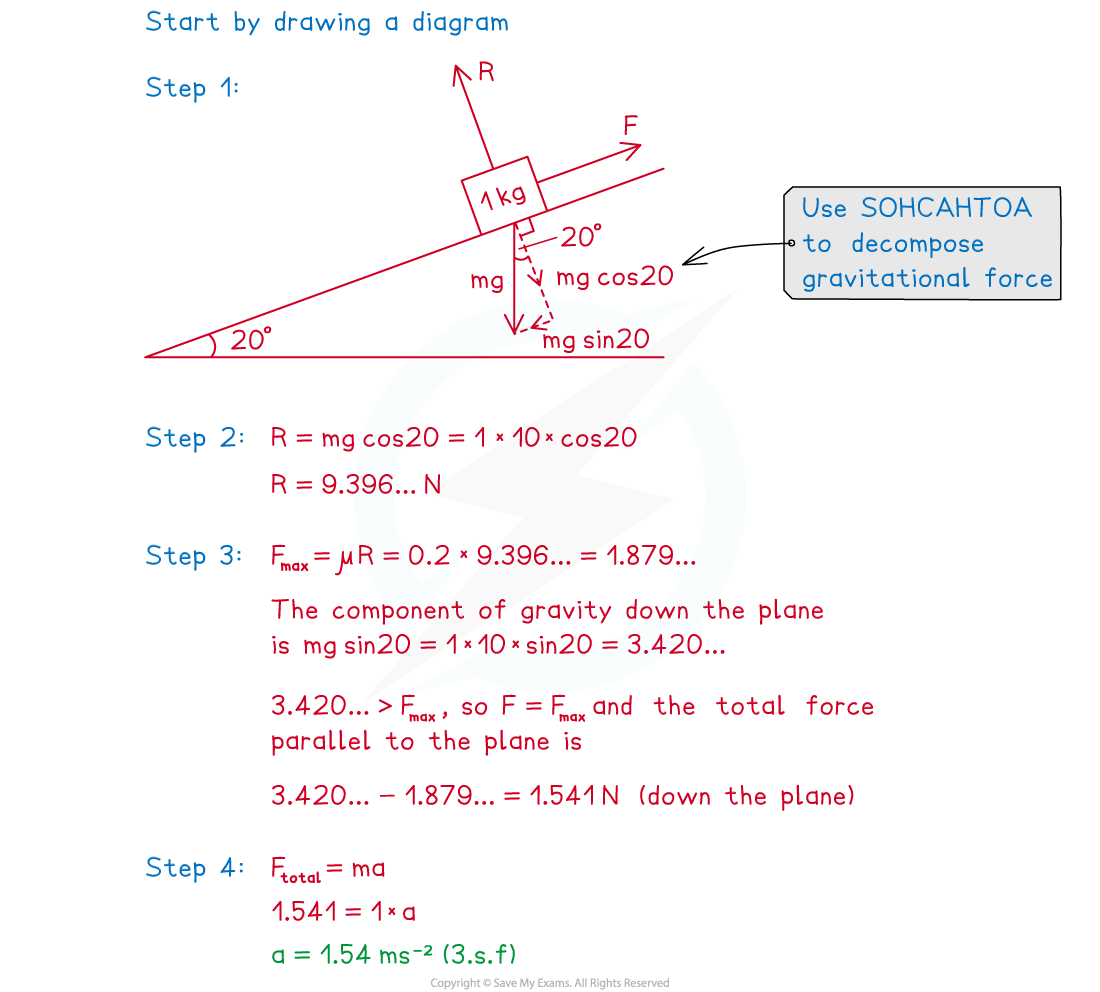# CIE A Level Maths: Mechanics复习笔记3.3.4 Coefficient of Friction - Inclined Planes

### Coefficient of Friction - Inclined Planes

#### How is the coefficient of friction used in problems involving inclined planes?

• On an inclined plane the basic principles are the same as for coefficient of friction on horizontal surfaces
• The important directions are parallel to the plane and perpendicular to the plane (instead of horizontal and vertical)

Step 1. Resolve weight (and any other forces if necessary) into components parallel and perpendicular to the plane

Step 2. Calculate the normal reaction force R

• Be careful – R will never simply be equal to mg in an inclined plane problem!
• R will always be directed perpendicular to and away from the plane
• the magnitude of R will always be such as to make the total perpendicular force zero

Step 3. Calculate FMAXμR and find the resultant (total force) of all the forces on the object that are parallel to the plane

• The force of friction will always act parallel to the plane in the direction opposite to any movement (or potential movement) of the object
• Remember – if the resultant of the other parallel forces is less than or equal to FMAX then friction will exactly balance those forces out and the object will remain stationary

Step 4. Use F = ma to determine the acceleration of the object

#### Worked Example

A wooden block of mass 1 kg is released from rest on a rough plane that is inclined at 20°  above the horizontal. The coefficient of friction between the block and the plane is 0.2. Find the acceleration of the block.#### Exam Tip

• Always draw a force diagram and label it clearly.
• Look out for the words smooth and rough in mechanics problems involving an object moving (or potentially moving) along a surface:
• If the surface is described as smooth then you can ignore friction in the problem (ie μ= 0)
• If the surface is described as rough than you need to include the force of friction in solving the problem
• If a friction question states that an object is on the point of moving that means that the object is in limiting equilibrium.